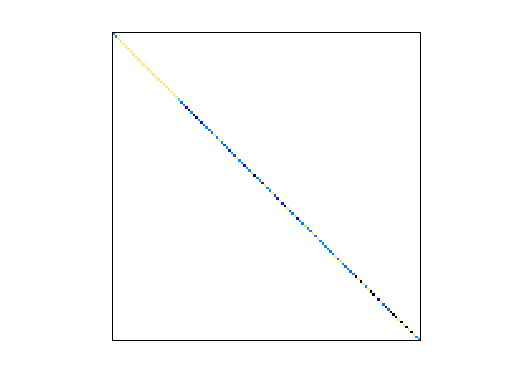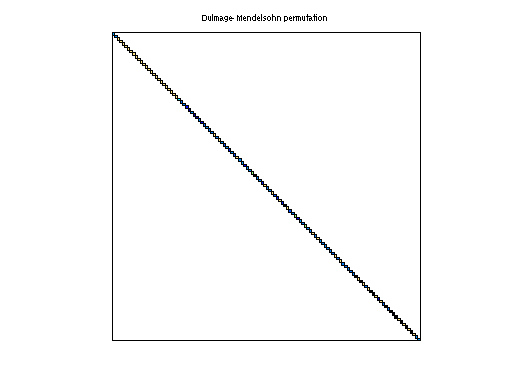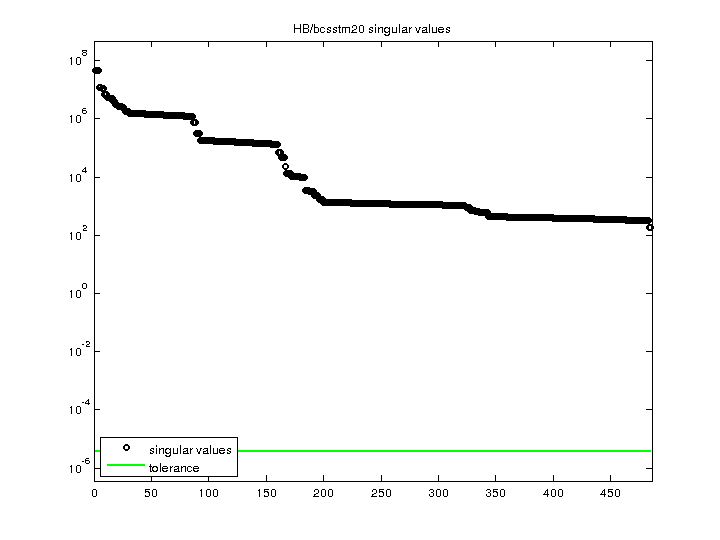Matrix: HB/bcsstm20

Description: SYMMETRIC MASS MATRIX - FRAME WITHIN A SUSPENSION BRIDGE(undirected graph drawing)• Matrix group: HB
• download as a MATLAB mat-file, file size: 3 KB. Use UFget(70) or UFget('HB/bcsstm20') in MATLAB.

 Matrix properties number of rows 485 number of columns 485 nonzeros 485 structural full rank? yes structural rank 485 # of blocks from dmperm 485 # strongly connected comp. 485 explicit zero entries 0 nonzero pattern symmetry symmetric numeric value symmetry symmetric type real structure symmetric Cholesky candidate? yes positive definite? yes

 author J. Lewis editor I. Duff, R. Grimes, J. Lewis date 1984 kind structural problem 2D/3D problem? yes

 Ordering statistics: result nnz(chol(P*(A+A'+s*I)*P')) with AMD 485 Cholesky flop count 4.8e+02 nnz(L+U), no partial pivoting, with AMD 485 nnz(V) for QR, upper bound nnz(L) for LU, with COLAMD 485 nnz(R) for QR, upper bound nnz(U) for LU, with COLAMD 485

 SVD-based statistics: norm(A) 4.77956e+07 min(svd(A)) 187.154 cond(A) 255381 rank(A) 485 sprank(A)-rank(A) 0 null space dimension 0 full numerical rank? yes

 singular values (MAT file): click here SVD method used: s = svd (full (A)) ; status: ok